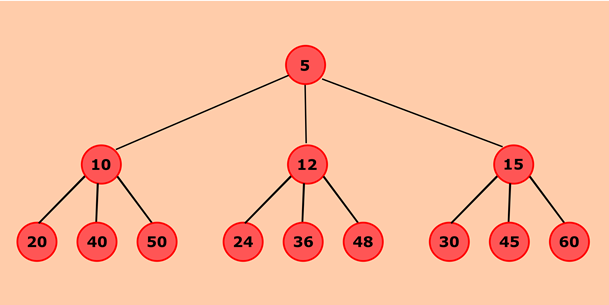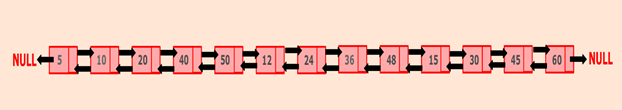Q:

# Java program to create a doubly linked list from a ternary tree

belongs to collection: Java Doubly Linked List Programs

In this program, the given ternary tree will be converted into a corresponding doubly linked list.

The ternary tree is a hierarchical data structure in which each node can have at most three children. This can be accomplished by traversing the ternary tree in a pre-order fashion that is, root -> left child -> middle child -> right child. First, traverse root node and add it to the list. Then, add its left, middle and right sub-trees respectively.

Ternary tree:### Algorithm

• Define a Node class which represents a node in the ternary tree. It will have four properties: data, left, middle, right where left, middle and right represent three children of a node.
• Root will represent the root of the ternary tree. Head and tail node represent the head and tail of the doubly linked list.
• convertTernaryToDLL() will convert the given ternary tree to corresponding doubly linked list.
• Nodes left, middle and right represent the child of given node.
• If the node is not null, then the node will be inserted into the list.
• If the list is empty, both head and tail will point to a node.
• If the list is not empty then, the node will be inserted at the end of the list. Here, pointer left, and right will represent the previous and next pointer of the doubly linked list. The middle will not point to anything hence, simply set it to null.
• When a node is successfully added into the list then, call convertTernaryToDLL() recursively to add a left, middle and right child of given node to the list.

a. displayDLL() will show all the nodes present in the list.

• Define a new node 'current' that will point to the head.
• Print current.data till current points to null.
• Current will point to the next node in the list in each iteration.

### Program:

``````public class TernaryTreeToDLL {

//Represent a node of ternary tree
public static class Node{
int data;
Node left;
Node middle;
Node right;

public Node(int data) {
this.data = data;
}
}

//Represent the root of the ternary tree
public Node root;
//convertTernaryToDLL() will convert the given ternary tree to corresponding doubly linked list
public void convertTernaryToDLL(Node node) {
//Checks whether node is null
if(node == null)
return;

//Keep three pointers to all three children
Node left = node.left;
Node middle = node.middle;
Node right = node.right;

//If list is empty then, add node as head of the list
//Both head and tail will point to node
node.middle = null;
//head's left will point to null
//tail's right will point to null, as it is the last node of the list
tail.right = null;
}
//Otherwise, add node to the end of the list
else {
//node will be added after tail such that tail's right will point to node
tail.right = node;
//node's left will point to tail
node.left = tail;
node.middle = null;
//node will become new tail
tail = node;
//As it is last node, tail's right will point to null
tail.right = null;
}

//Add left child of current node to the list
convertTernaryToDLL(left);
//Then, add middle child of current node to the list
convertTernaryToDLL(middle);
//Then, add right child of current node to the list
convertTernaryToDLL(right);
}

//displayDLL() will print out the nodes of the list
public void displayDLL() {
//Node current will point to head
System.out.println("List is empty");
return;
}
System.out.println("Nodes of generated doubly linked list: ");
while(current != null) {
//Prints each node by incrementing the pointer.

System.out.print(current.data + " ");
current = current.right;
}
System.out.println();
}

public static void main(String[] args) {

TernaryTreeToDLL tree = new TernaryTreeToDLL();
//Add nodes to the ternary tree
tree.root = new Node(5);
tree.root.left = new Node(10);
tree.root.middle = new Node(12);
tree.root.right = new Node(15);
tree.root.left.left = new Node(20);
tree.root.left.middle = new Node(40);
tree.root.left.right = new Node(50);
tree.root.middle.left = new Node(24);
tree.root.middle.middle = new Node(36);
tree.root.middle.right = new Node(48);
tree.root.right.left = new Node(30);
tree.root.right.middle = new Node(45);
tree.root.right.right = new Node(60);

//Converts the given ternary tree to doubly linked list
tree.convertTernaryToDLL(tree.root);

//Displays the nodes present in the list
tree.displayDLL();
}
}  ``````

Output:

```Nodes of generated doubly linked list:
5 10 20 40 50 12 24 36 48 15 30 45 60```